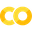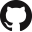# Use TSDataset and Forecaster for Time-Series Forecasting¶Run in Google ColabView source on GitHub

In this guide we will demonstrate how to use Chronos TSDataset and Chronos Forecaster for time seires processing and forecasting in 4 simple steps.

## Step 0: Prepare Environment¶

We recommend using conda to prepare the environment. Please refer to the install guide for more details.

```conda create -n zoo python=3.7 # "zoo" is conda environment name, you can use any name you like.
conda activate zoo
pip install analytics-zoo[automl] # install latest nightly build
```

## Step 1: Data transformation and feature engineering using Chronos TSDataset¶

TSDataset is our abstract of time series dataset for data transformation and feature engineering. Here we use it to preprocess the data.

Initialize train, valid and test tsdataset from raw pandas dataframe.

```from zoo.chronos.data import TSDataset
from sklearn.preprocessing import StandardScaler

tsdata_train, tsdata_valid, tsdata_test = TSDataset.from_pandas(df, dt_col="timestamp", target_col="value",
with_split=True, val_ratio=0.1, test_ratio=0.1)
```

Preprocess the datasets. Here we perform:

• deduplicate: remove those identical data records

• impute: fill the missing values

• gen_dt_feature: generate feature from datetime (e.g. month, day…)

• scale: scale each feature to standard distribution.

• roll: sample the data with sliding window.

• For forecasting task, we will look back 3 hours’ historical data (6 records) and predict the value of next 30 miniutes (1 records).

We perform the same transformation processes on train, valid and test set.

```lookback, horizon = 6, 1

scaler = StandardScaler()
for tsdata in [tsdata_train, tsdata_valid, tsdata_test]:
tsdata.deduplicate().impute().gen_dt_feature()\
.scale(scaler, fit=(tsdata is tsdata_train))\
.roll(lookback=lookback, horizon=horizon)
```

## Step 2: Time series forecasting using Chronos Forecaster¶

After preprocessing the datasets. We can use Chronos Forecaster to handle the forecasting tasks.

Transform TSDataset to sampled numpy ndarray and feed them to forecaster.

```x, y = tsdata_train.to_numpy()
x_val, y_val = tsdata_valid.to_numpy()
# x.shape = (num of sample, lookback, num of input feature)
# y.shape = (num of sample, horizon, num of output feature)

forecaster = TCNForecaster(past_seq_len=lookback,  # number of steps to look back
future_seq_len=horizon,  # number of steps to predict
input_feature_num=x.shape[-1],  # number of feature to use
output_feature_num=y.shape[-1])  # number of feature to predict
res = forecaster.fit((x, y), validation_data=(x_val, y_val), epochs=3)
```

## Step 3: Further deployment with fitted forecaster¶

Use fitted forecaster to predict test data

```x_test, y_test = tsdata_test.to_numpy()
pred = forecaster.predict(x_test)
pred_unscale, groundtruth_unscale = tsdata_test.unscale_numpy(pred), tsdata_test.unscale_numpy(y_test)
```

Save & restore the forecaster.

```forecaster.save("nyc_taxi.fxt")
forecaster.restore("nyc_taxi.fxt")
```